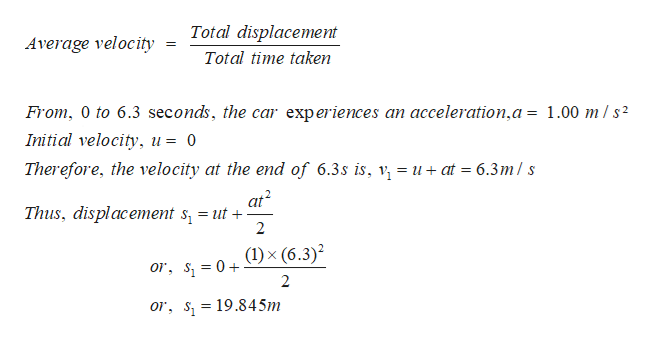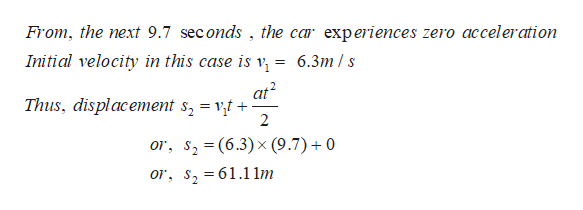An car initially at rest experiences an acceleration of 1.00 m/s² for 6.30 s then travels at that constant velocity for another 9.70 s. What is the magnitude of the object’s average velocity over the 16 s interval?

Question

An car initially at rest experiences an acceleration of 1.00 m/s² for 6.30 s then travels at that constant velocity for another 9.70 s. What is the magnitude of the object’s average velocity over the 16 s interval?

Step 1

Average velocity is :help_outlineImage TranscriptioncloseTotal displacement Average velocity Total time taken From, 0 to 6.3 seconds, the car experiences an acceleration,a = 1.00 m/s2 Initial velocity, u= 0 Therefore, the velocity at the end of 6.3s is, v, = u+ at = 6.3m/s at2 Thus, displacement s = ut + 2 0, s =0+x (6.3)2 2 or, S 19.84 5m fullscreen
Step 2

Further,...help_outlineImage TranscriptioncloseFrom, the next 9.7 seconds , the car experiences zero acceleration Initial velocity in this case is v 6.3m/s at2 Thus, displacement s, = vt 2 0r, s2 (6.3) x (9.7)0 or, s 61.1 1m fullscreen

Want to see the full answer?

See Solution

Want to see this answer and more?

Our solutions are written by experts, many with advanced degrees, and available 24/7

See Solution
Tagged in

Other